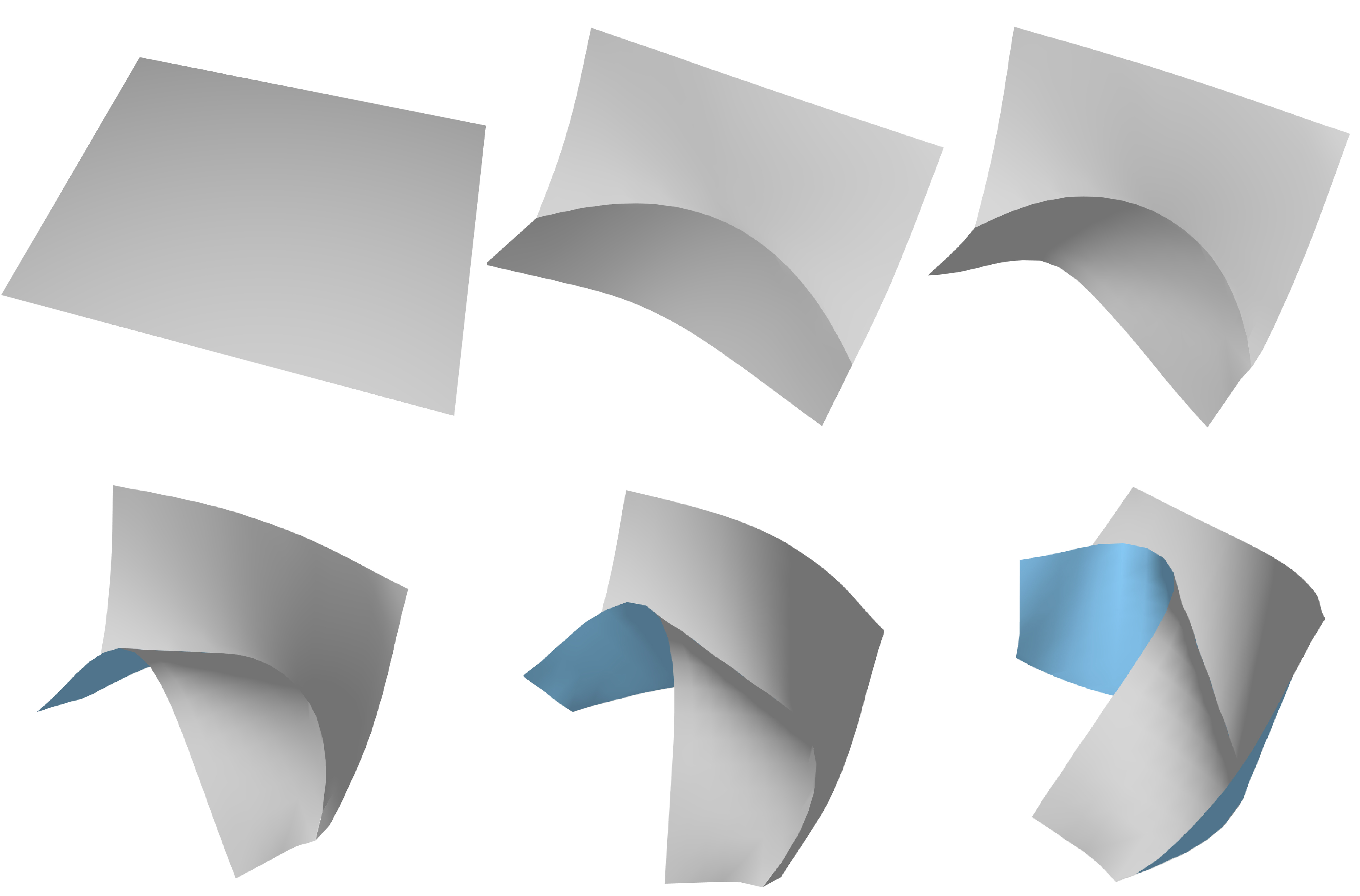## The Shape Space of Discrete Orthogonal Geodesic Nets

publication
ACM SIGGRAPH ASIA 2018
authors
Michael Rabinovich, Tim Hoffmann, Olga Sorkine-HornungWe develop a theory for shape space exploration of DOG nets, which are discrete developable surfaces parameterized by orthogonal geodesics. Our study results in a characterization of this shape space and an algorithm to discretize smooth flows on DOGs, as used in the above animation.

### abstract

Discrete orthogonal geodesic nets (DOGs) are a quad mesh analogue of developable surfaces. In this work we study continuous deformations on these discrete objects. Our main theoretical contribution is the characterization of the shape space of DOGs for a given net connectivity. We show that generally, this space is locally a manifold of a fixed dimension, apart from a set of singularities, implying that DOGs are continuously deformable. Smooth flows can be constructed by a smooth choice of vectors on the manifold's tangent spaces, selected to minimize a desired objective function under a given metric. We show how to compute such vectors by solving a linear system, and we use our findings to devise a geometrically meaningful way to handle singular points. We base our shape space metric on a novel DOG Laplacian operator, which is proved to converge under sampling of an analytical orthogonal geodesic net. We further show how to extend the shape space of DOGs by supporting creases and curved folds and apply the developed tools in an editing system for developable surfaces that supports arbitrary bending, stretching, cutting, (curved) folds, as well as smoothing and subdivision operations.